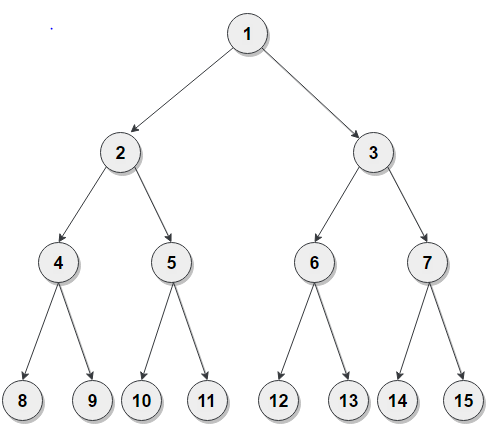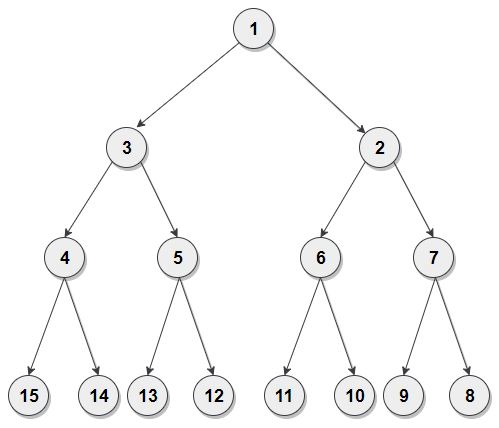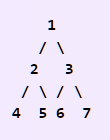# Reverse Alternate Nodes

Posted: 8 Oct, 2020
Difficulty: Moderate

## PROBLEM STATEMENT

#### A perfect binary tree is a binary tree in which all the interior nodes have two children, and all leaves have the same depth or same level.

##### Example :
``````Given binary tree :
````````````After the reversal of alternate nodes, the tree will be :
``````##### Input Format :
``````The first line contains elements of the given binary tree in the level order form. The line consists of values of nodes separated by a single space. In case a node is null, we take -1 in its place.

For example, the input for the tree depicted in the below image would be :
````````````1
2 3
4 5 6 7
-1 -1 -1 -1 -1 -1 -1 -1
``````

#### Explanation :

``````Level 1 :
The root node of the tree is 1

Level 2 :
Left child of 1 = 2
Right child of 1 = 3

Level 3 :
Left child of 2 = 4
Right child of 2 = 5
Left child of 3 = 6
Right child of 3 = 7

Level 4 :
Left child of 4 = null (-1)
Right child of 4 = null (-1)
Left child of 5 = null (-1)
Right child of 5 = null (-1)
Left child of 6 = null (-1)
Right child of 6 = null (-1)
Left child of 7 = null (-1)
Right child of 7 = null (-1)

The first not-null node(of the previous level) is treated as the parent of the first two nodes of the current level. The second not-null node (of the previous level) is treated as the parent node for the next two nodes of the current level and so on.
The input ends when all nodes at the last level are null(-1).
``````
##### Note :
``````The above format was just to provide clarity on how the input is formed for a given tree.
The sequence will be put together in a single line separated by a single space. Hence, for the above-depicted tree, the input will be given as :

1 2 3 4 5 6 7 -1 -1 -1 -1 -1 -1 -1 -1
``````
##### Output Format :
``````Print in a single line 'N' single space-separated integers denoting the inorder traversal of the modified tree.
``````
##### Note :
``````You do not need to print anything. It has already been taken care of, just implement the given function.
``````
##### Constraints :
``````0 <= N <= 5*10^5
0 <= node.data <= 10^7 and node.data != -1

Time Limit : 1 sec
``````Approach 1

The idea is to perform level order traversal of the given perfect binary tree and traverse its nodes level by level. If the current level is even then, we will reverse all node values for the current level. For the even level, we will push all nodes which are present at the current level into the queue and correspond the value of that node into the stack. And once we are done with the current level, we will get each node one by one from the queue and set the value of that node from the stack. So in this way, the values of all the nodes will reverse for the current level.

Here is the algorithm :

1. We will be using the queue for level order traversal, let’s say, ‘PENDING’. Initially, push the root node into the ‘PENIDNG’.
2. Now we will go for each level, and if any current level becomes even, we will reverse all the values of nodes for the current level. Steps are as follows :
• Push all nodes for the current level into the queue; let’s say, ‘levelNodes’.
• Push all the values corresponding to the node into the stack, let’s say, ‘levelValues’.
• Once we are done with the current level, we will be fetching one by one node from ‘levelNodes’ and make the value of that node corresponding to the top of ‘levelValues’.
3. Finally, return the root of the tree after complete the level order traversal of the Tree.